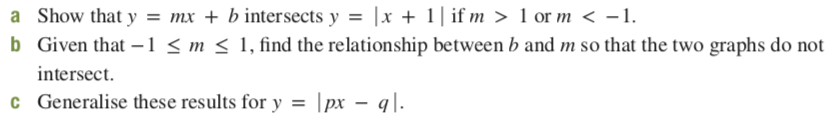# a Show that y = mx + b intersects y = |x + 1| if m > 1 or m < -1.b Given that -1 < m < 1, find the relationship between b and m so that the two graphs do notintersect.c Generalise these results for y = |px – q|.

Question
72 viewshelp_outlineImage Transcriptionclosea Show that y = mx + b intersects y = |x + 1| if m > 1 or m < -1. b Given that -1 < m < 1, find the relationship between b and m so that the two graphs do not intersect. c Generalise these results for y = |px – q|. fullscreen
check_circle

Step 1

Given,

y = mx + c

For m > 1

Take  m = 2 > 1,

Step 2

Sketch the graph of y = 2x + c and y = |x + 1 | in the following.

Step 3

This shows that, both functions are intersect at (-1.333,0.333).

For m < - 1

Take  m = - 2 < - 1,

Sketch the graph of y...

### Want to see the full answer?

See Solution

#### Want to see this answer and more?

Solutions are written by subject experts who are available 24/7. Questions are typically answered within 1 hour.*

See Solution
*Response times may vary by subject and question.
Tagged in

### Math